## C Examples

The best way to learn C programming is by practicing lots of example programs. The benefit of practicing examples, besides learning concepts is improved problem-solving skills and coding skills. Some programs in the list given below include flowchart to help you understand the program logic. We highly recommend trying these examples on your own, before comparing the solutions.

### Prerequisites

The prerequisites to learn and practice these examples listed below.

• C Tutorial – learn C programming language before you practice example programs.
• C Compiler – you will need a C compiler to run these example programs. Learn how to install Turbo C++ 3.0 compiler.
• Pen and Paper – Some programs especially math related programs require you to verify the output manually.

### C Examples

Here is a list of example C programs.

## C Program to Reverse a Number using Recursion

The program uses recursion to reverse an input number. A function is recursively called to extract each digit from the number and place it in reverse order. The final output is a reversed number. We wrote the program using Dev C++ version 4.9.9.2 on a Windows 7 64-bit system.

You must be familiar with following c programming concept before working on the example.

### Problem Definition

The program receives an input number and reverse each by extracting the digits one at a time from original number n. It starts with two initial conditions. The remainder r = 0 and if n=0 then return 0.

The procedure to reverse the number 567 is shown below. The program does the following tasks to reverse the input number.

1. Extract a digit from the unit position.
2. Each iteration multiply the number with 10 to reverse it.
3. Next extracted digit is added to previously obtained result.
4. After final extraction the number is completely reversed.

Go through each step below to understand the working of the reverse() function.

Step 1: Extract the number 7

\begin{aligned}&r = r + n \hspace{2mm}\% \hspace{2mm}10\\ \\
&r = 0 + 567 \hspace{2mm}\% \hspace{2mm}10\\ \\
&t = 7
\end{aligned}

Step 2: Change the position of a number from unit place to tenth place.

\begin{aligned}
&r = r * 10\\ \\
& r = 7 * 10\\ \\
&r = 70
\end{aligned}

Step 3: Get ready for the next extraction.

\begin{aligned}
&= reverse (n/10)\\ \\
&= reverse (567/10)\\ \\
&= reverse (56)
\end{aligned}

Step 4: Extraction the second digit when value of r = 70 from Step 2.

\begin{aligned}
&r = 70 + n \% 10\\ \\
&r = 70 + 56 \% 10\\ \\
&r = 70 + 6\\ \\
&r = 76
\end{aligned}

Step 5: Change position of r to tenth place to hundredth place.

\begin{aligned} &r = r * 10\\ \\
&r = 76 * 10\\ \\
&r = 760
\end{aligned}

Step 6: Get ready for a new n value.

\begin{aligned}
&= reverse (n/10)\\ \\
& = reverse ( 56/1)\\ \\
& = reverse (5)
\end{aligned}

Step 7: Extract last digit from original number, when r = 760

\begin{aligned}
&r = r + n \% 10\\ \\
& r = 760 + 5\\ \\
&r = 765
\end{aligned}

The number is successfully reversed.

Note:- The above steps are demonstration of reversing a 3 digit number, hence, the number of steps are limited. If you take a larger number there will be more steps.

### Program Code – Program to reverse a number using recursion

#include
#include
int reverse(int);
int main()
{
int n, r, i;
printf("Enter a Number to Reverse:");
scanf("%d", & n);
r = reverse(n);
for (i = 0; i < 30; i++)
printf("_"); printf("\n\n");
printf("%d\n\n", r);
for (i = 0; i < 30; i++)
printf("_"); printf("\n\n");
system("PAUSE");
return (0);
}

/* The Reverse Function */

int reverse(int n)
{
static long r = 0;
if (n == 0)
{
return 0;
}
r = r * 10;
r = r + n % 10;
reverse(n / 10);
return r;
}

### Output

The program ask for an input number, when you enter a number – for example, 244. The program reverses the digits of the number and prints on the console. The solution is 442.

Enter a Number to Reverse: 244
_______________________________
442
_______________________________

## C Program To Identify A Quadrilateral

The program to identify a quadrilateral read inputs – length of sides and angles made by the quadrilateral shape and prints the shape name as output.

We compiled the program using Dev-C++ compiler installed on a windows 7 64-bit system. You may try another standard C compiler, but make sure to modify the code according to your compiler specifications.

You must be aware of following C programming concept to understand this example program.

Before you practice this program, you must go through the concepts of quadrilateral shapes and their differences. See the problem definition section.

### Problem Definition

A quadrilateral is a polygon shape that has 4 sides and corners that make 4 angles. The sum of those angles must be equal to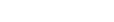. There are many types of quadrilateral shapes, you will find a brief description of each shape below.

Square

A square has 4 equal sides and 4 right angles at each corner.

Rectangle

A rectangle has 4 sides with parallel sides are of the same length and 4 corners are right angles. The rectangle has a longer side and a shorter side.

Rhombus

A rhombus is like a square or diamond with 4 equal sides, but none of the corners is a right angle.

Parallelogram

Any quadrilateral that has a pair of parallel sides is a parallelogram. There are shapes which demonstrate properties of parallelogram – square, rectangle, and a rhombus are special case of a parallelogram.

Trapezoid

If a quadrilateral contains at least one pair of parallel lines then it is called a trapezoid.

Kite

A kite is a quadrilateral that has a pair of adjacent sides of equal length and the angle where these two pair meet are equal.

### Program Code

#include <stdio.h>
#include <stdlib.h>
int main()
{
float a, b, c, d, sum = 0.0;
float angle, arr, len, tmp; int i;
int flag, square, rectangle, rhombus, para, trap;
flag = square = rectangle = rhombus = trap = 0;
para = 0;
printf("Enter length of all sides of the Quadrilateral:");
scanf("%f", & arr);
scanf("%f", & arr);
scanf("%f", & arr);
scanf("%f", & arr);
len = arr;
printf("Enter Angles of all Corners of the Quadrilateral:");
for (i = 0; i < 4; i++)
{
scanf("%f", & angle[i]);
if (angle[i] == 90)
{
flag++;
}
}

/* 360 degree check */
for (i = 0; i < 4; i++)
{
sum = sum + angle[i];
}
if (sum == 360)
{
printf("sum of angle is 360 n");
}
else
{
printf("Sum of Angles is not 360:n");
abort();
}

/* Identify Square */
for (i = 0; i < 4; i++)
{
if (arr[i] == len)
{
square++;

if (square == 4 && flag == 4)
{
for (i = 0; i < 30; i++)
printf("_"); printf("\n\n");
printf("It is a Square:\n\n");
for (i = 0; i < 30; i++)
printf("_"); printf("\n\n");
}
}
}

/* Identify rectangle */
if (arr == arr && arr == arr && arr != arr && flag == 4)
{
for (i = 0; i < 30; i++)
printf("_"); printf("\n\n");
printf("It is a Rectangle:\n\n");
for (i = 0; i < 30; i++)
printf("_"); printf("\n\n");
}

/* identify parallelogram */
for (i = 0; i < 4; i++)
{
if (angle[i] == 90)
{
para++;
}
}
if (arr == arr || arr == arr)
{
if (para < 4)
{
for (i = 0; i < 30; i++)
printf("_"); printf("\n\n");
printf("It is a Parallelogram :\n\n");
for (i = 0; i < 30; i++)
printf("_"); printf("\n\n");
}
}

/* Identify Kites */
if (arr == arr || arr == arr)
{
if (para < 3 && angle == angle && angle == angle)
{
if (arr != arr)
for (i = 0; i < 30; i++)
printf("_"); printf("\n\n");
printf("It is a Kite :\n\n");
for (i = 0; i < 30; i++)
printf("_"); printf("\n\n");
}
}

/* Identify rhombus */
for (i = 0; i < 4; i++)
{
if (arr[i] == len)
{
rhombus++;
if (rhombus == 4 && angle[i] != 90)
{
for (i = 0; i < 30; i++)
printf("_"); printf("\n\n");
printf("It is a Rhombus:\n\n");
for (i = 0; i < 30; i++)
printf("_"); printf("\n\n");
}
}
}

/* Identify trapezoid */
for (i = 0; i < 4; i++)
{
len = arr[i];
tmp = angle[i];
for (i = 0; i < 4; i++)
{
if (arr[i + 1] != len && angle[i] != tmp)
{
trap++;
}
}
}

if (trap == 3)
{
for (i = 0; i < 30; i++)
printf("_"); printf("\n\n");
printf("It is a Trapezoid!\n");
for (i = 0; i < 30; i++)
printf("_"); printf("\n\n");
}
system("PAUSE");
return 0;
}

### Output

Enter length of all sides of the Quadrilateral:20
20
20
20
Enter Angles of all Corners of the Quadrilateral:90
90
90
90
sum of angle is 360
________________________________
It is a Square:
________________________________
Enter length of all sides of the Quadrilateral:12
12
12
12
Enter Angles of all Corners of the Quadrilateral:60
120
60
120
sum of angle is 360
____________________________
It is a Parallelogram :
____________________________
____________________________
It is a Rhombus:
____________________________

## C Program For Law Of Sine Problems

With the law of sine, you can find any unknown angle of a given triangle or the length of a particular side of a triangle or the length of a particular side of a triangle. This is a fundamental concept of trigonometry.

We used Dev-C++ to compile the program, but you may use any other standard C compiler. This program make use of math header – math.h  especially, two trig functions – sin () function and asin () function at lot. So if you are choosing a different compiler then use the correct math header file.

Before you try the example, learn following C programming concepts. You can skip it if already know it well.

### Problem Definition

The law of sine is given below. The triangle has three sides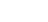and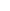; It also has three angles –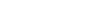and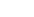.

In general, there are two cases for problems involving the law of sine.

#### Case 1: When the length of two sides are given and the angle opposite to one of the sides with length is given.

When the length of two side –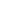andare given and the angle opposite to sideis given. Then using law of sine

\begin{aligned}&X \hspace{1ex} = \hspace{1ex} sin \hspace{1ex} \frac{A}{a}\\ \\
&sin  \hspace{1ex}\frac{B}{b} \hspace{1ex} = \hspace{1ex} X\\ \\
&sin \hspace{1ex} B \hspace{1ex} =\hspace{1ex}  X \hspace{1ex}\ast \hspace{1ex}b\\ \\
&\angle {B }\hspace{1ex} = \hspace{1ex} arcsin ( X \ast b)
\end{aligned}

This the way to find the value of sinand then using arcsin to find the.

#### Case 2: When 2 angles – angle A and angle B are given with length of the side opposite to angle A or angle B.

When two angles and length of at least one side opposite to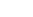oris given. Find the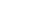and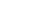values first.

Find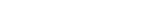or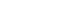, whichever is given in the problem.

The length of side

\begin{aligned}b \hspace{1ex} = \hspace{1ex} sin\hspace{2px} B \cdot X\end{aligned}

This is how you will find the value of the length of side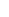whenandis given along with the length of side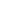.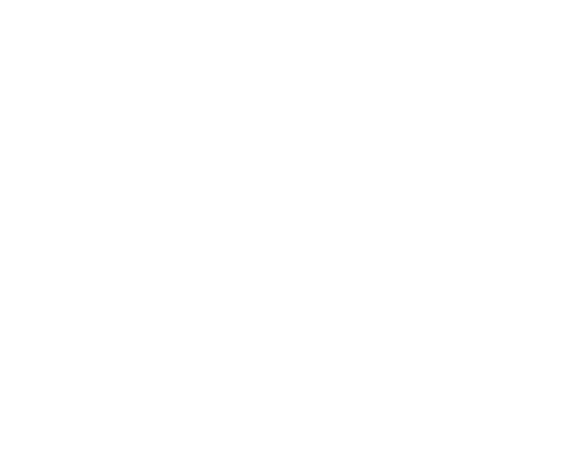Figure 3: Two Angles and 1 Side for Law of Sine Problems

In next section, you will find the flowchart of the program for the law of sine and the above two cases to understand the logic of the program.

### Flowchart – Program for Law of Sine Problems

The next flowchart is of function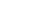which covers the case 1 of the law of sine problems.

The flowchart for functionthat covers the case 2 of the law of sine problems is given below.

### Program Code – Program for Law of Sine Problems

/* C Program for solving law of sine problems */
#include <stdio.h>
#include <math.h>
#include<stdlib.h>
/* Variable declarations */
int ch,i;
float a, b, q, p, deg;
float angle,angle2, res;
/* Function declaration */
void s1();
void s2();
int main()
{
for(i=0;i < 30;i++)
printf("*");printf("\n\n");
for(i=0;i < 30;i++)
printf("*");printf("\n\n");
printf("1.Sine Problem with 2 Sides and 1 Angle:\n");
printf("2.Sine Problem with 2 Angles and 1 Side:\n");
printf("3.Quit using any other number:\n\n");
for(i=0;i < 30;i++)
printf("*");printf("\n\n");
while(1)
{
scanf("%d",& ch);
if(ch == 1)
{
s1();
}
else if(ch == 2)
{
s2();
}
else if(ch == 3)
{
exit(0);
}
else
{
printf("Wrong choice ! Try again:\n");
}
}
system("PAUSE");
return 0;
}
/* Main function ends */
/* Function definition - s1() */
void s1()
{
float pi = 3.141;
for(i=0;i < 30;i++)
printf("_");printf("\n\n");
printf("Enter value for side with angle:");
scanf("%f",&a);
printf("Enter value for side without angle:");
scanf("%f",&b);
printf("Enter the angle(degrees):");
scanf("%f",&angle);
for(i=0;i < 30;i++)
printf("_");printf("\n\n");
angle = angle * (pi/180);
q = sin(angle);
p = b * (q/a);
deg = asin(p);
res = deg * (180/pi);
for(i=0;i < 30;i++)
printf("_");printf("\n\n");
printf("Angle B = %f\n",res);
for(i=0;i < 30;i++)
printf("_");printf("\n\n");
}
/* Function definition - s2() */
void s2()
{
float pi = 3.141;
for(i=0;i < 30;i++)
printf("_");printf("\n\n");
printf("Enter value of side a with angle:");
scanf("%f",&a);
printf("Enter angle A for side a:");
scanf("%f",&angle);
printf("Enter value angle B of side b:");
scanf("%f",&angle2);
for(i=0;i < 30;i++)
printf("_");printf("\n\n");
angle = angle * (pi/180);
angle2 = angle2 * (pi/180);
q = sin(angle);
p = sin(angle2);
deg = q/a;
b = p/deg;
for(i=0;i < 30;i++)
printf("_");printf("\n\n");
printf("Side b = %f\n",b);
for(i=0;i<30;i++)
printf("_");printf("\n\n");
}

### Outputs from the Program

The program gives three choices during run time

1. Apply the law of sine when the length of 2 sides and 1 angle opposite to a given side is available.
2. Apply the law of sine when 2 angles are given and length of 1 side opposite to an angle is given.
3. Quit the program

The output from both the cases are given below, you should run the program and verify the output for yourself.

                                Menu
***********************************************************************
1.Sine Problem with 2 Sides and 1 Angle:
2.Sine Problem with 2 Angles and 1 Side:
3.Quit using any other number:
***********************************************************************
_______________________________________________________________________
Enter value for side with angle:5
Enter value for side without angle:4
Enter the angle(degrees):60
_______________________________________________________________________
_______________________________________________________________________
Angle B = 43.855770
_______________________________________________________________________
Enter your Choice:_

Now, we will test option 2 from the menu.

                                Menu
***********************************************************************
1.Sine Problem with 2 Sides and 1 Angle:
2.Sine Problem with 2 Angles and 1 Side:
3.Quit using any other number:
***********************************************************************
_______________________________________________________________________
Enter value of side a with angle:30
Enter angle A for side a:14
Enter value angle B of side b:55
_______________________________________________________________________
_______________________________________________________________________
Side b = 101.586449
_______________________________________________________________________
Enter your Choice:_

## C Program For A Simple Calculator

This example program accepts two input numbers and performs an arithmetic operation such as addition, subtraction, division, multiplication and mod operation on them. The output is printed to the console.

Learn following C programming concepts before trying this example on your computer.

### Program Definition

This calculator program is built using C arithmetic operators. The user is presented with a list of choices. Once the user input his or her choice that operation is performed.

Here is the list of operations presented to the users.

2. Subtraction
3. Multiplication
4. Division
5. Modulo
6. Close

The C calculator is implemented using the switch-case mechanism of C language.

### Program Code

/* Program for a simple calculator
in C using Switch_Case */
#include <stdio.h>
#include <conio.h>
main()
{
/* Variable declarations */
int i,a,b,choice,result;
printf("ENTER TWO NUMBERS:n");
printf("a=:");
scanf("%d",&a);
printf("b=:");
scanf("%d",&b);
i=0;
while(choice != 6)
{
i++;
}
getch();
return 0;
}
void menu(int a, int b, int choice)
{
int result,i;
for(i=0;i < 35;i++)
printf("*"); printf("\n\n");
printf("\tC CALCULATOR\n\n");
for(i=0;i < 35;i++)
printf("_"); printf("\n\n");
printf("2.SUBTRACTION\n");
printf("3.MULTIPLICATION\n");
printf("4.DIVISION\n");
printf("5.MODULO \n");
printf("6.CLOSE\n");
for(i=0;i < 35;i++)
printf("_"); printf("\n\n");
scanf("%d",&choice);
switch(choice)
{
case 1:
result = a + b;
for(i=0;i < 35;i++)
printf("_"); printf("\n\n");
printf("Result=%d\n",result);
printf("\n\n");
break;
case 2:
result = a - b;
for(i=0;i < 35;i++)
printf("_"); printf("\n\n");
printf("Result=%d\n",result);
/* Subtraction */
printf("\n\n");
break;
case 3:
result = a * b;
for(i=0;i < 35;i++)
printf("_"); printf("\n\n");
printf("Result=%d\n",result);
/* Multiplication */
break;
case 4:
result = a/b;
for(i=0;i < 35;i++)
printf("_"); printf("\n\n");
printf("Result=%d\n",result);
/* Division operation */
printf("\n\n");
break;
case 5:
result = a % b;
for(i=0;i < 35;i++)
printf("_"); printf("\n\n");
printf("Result=%d\n",result);
/* Modulo operation */
printf("\n\n");
break;
default:
exit(0);
}
}

Each arithmetic operator performs their respective operation on user inputs and return the results. Here is the list of important expressions used in the program.

result = a + b;
result = a - b;
result = a * b;
result = a / b;
result = a % b;

### Output

The calculator program shows options when you run it. Enter two input numbers and then enter your choice of arithmetic operation.

ENTER TWO NUMBERS:
a=:33
b=:55
****************************************
C CALCULATOR
________________________________________
2.SUBTRACTION
3.MULTIPLICATION
4.DIVISION
5.MODULO
6.CLOSE
________________________________________

_

The output will be displayed on the screen.

                C CALCULATOR
___________________________________________________
2.SUBTRACTION
3.MULTIPLICATION
4.DIVISION
5.MODULO
6.CLOSE
___________________________________________________
1
___________________________________________________
Result=88

## C Program To Count Frequency Of Vowels

The C program to count the frequency of vowels, consonants and white spaces for a given word or a sentence is a string manipulation program. A C loop with conditions that checks the given input string and count the number of vowels, consonants, and white spaces.

We compiled this program using Dev-C++ compiler installed on a Windows 7 64-bit system. You may try another standard C compiler and to get an error free program make necessary changes to the source code.

Also, before you try this example, learn following C programming concepts. If you are familiar with them, continue reading.

### Problem Definition

In C language every string is like an array with each character of the string hold a position in this array. The last character is null character by default.

Using this array logic, the program read a word or a sentence during execution and check each and every character of input string. It counts the number of vowels, consonants and white spaces.

For example, the array representation of word – SPIDERMAN as follows.

\begin{aligned} &0 \hspace{5px}1  \hspace{5px}2\hspace{5px} 3 \hspace{5px}4 \hspace{5px}5 \hspace{5px}6\hspace{5px} 7\hspace{5px} 8 \hspace{5px}9 \\
&S \hspace{3px} P\hspace{3px} I \hspace{3px}D \hspace{3px}E \hspace{3px}R \hspace{3px}M\hspace{3px} A \hspace{3px}N \hspace{3px}\backslash\empty\end{aligned}

Note:- The Null character is added to all the strings in C by default.

### Program Code – Program to Count Frequency of Vowels

/*Read a sentence and print frequency of each vowels and the total count of consonants */
#include <stdio.h>
#include <conio.h>
int main()
{
char str;
int a,e,i,o,u,consonant,whitespace;
a = e = i = o = u = consonant = whitespace = 0;
int k;
/* Get the Sentence */
gets(str);
for(k=0;k<strlen(str);k++)
{
if(str[k]== 'a')
{
a = a+1;
}
else if(str[k] == 'e')
{
e = e +1;
}
else if(str[k] == 'i')
{
i = i +1;
}
else if( str[k] == 'o')
{
o = o + 1;
}
else if(str[k] == 'u')
{
u = u+1;
}
else if((str[k] >= 'a' && str[k] <= 'z')||(str[k] >= 'A' && str[k] <= 'Z'))
{
consonant ++;
}
else if (str[k] == ' ')
{
whitespace++;
}
}
printf("a :%d\n",a);
printf("e :%d\n",e);
printf("i :%d\n",i);
printf("o :%d\n",o);
printf("u :%d\n",u);
printf("Consonant :%d\n",consonant);
printf("whitespace :%d\n",whitespace);
/* count vowels and consonants */
/* print the results */
system("PAUSE");
return 0;
}

Apart from being a string program, we used built-in functions from C standard library to get string input (gets) and compute the length of the given input string (strlen).

### Output

The output of the program is given below. When the sentence ” A large reptile” is entered, the program reads all the vowels, consonants and white spaces and print the results.

ENTER A WORD OR A SENTENCE:
A large reptile
_____________________________________________
a :1
e :3
i :1
o :0
u :0

CONSONANTS :8
WHITESPACES :2

## C Program To Display Student Results

The C program to display student results demonstrates the working of conditional statement in the C language. The program takes student’s marks in percentage (%) as input , process the input value and displays the results (pass or fail) as output.

The output depends on the conditional statement in the example program.

Learn C programming basics before you begin with this example program.

### Problem Definition

The program decides whether a student has passed or failed an exam. If the input is in percentage mark ( say 70%), a simple conditional statement checks if given percentage mark is below 40%.

( mark < 40)

The student result is a pass when mark is above 40% , else the student has failed. The result is displayed at the console.Here we list the steps involved in processing input values.

1. Receive input in percentage mark (%)
2. Check if the given mark is above below 40%
3. If below 40%, then the student has failed
4. Else the student passed.
5. Display the result.

### Flowchart – Program to Display Student Results

The program start at the top (Start) and terminates where it says, (End) in the flowchart.

### Program Code – Program to Display Student Results

/*Show result of student using Marks */
#include <stdio.h>
#include <conio.h>
int main()
{
int marks,i;
printf("Enter the marks (in percentage ) of Student:");
scanf("%d",&marks);
/* display result */
if(marks < 40)
{
for(i=0;i<40;i++)
printf("_");printf("\n\n");
printf("Result = Failed\n");
for(i=0;i<40;i++)
printf("_");printf("\n\n");
}
else
{
for(i=0;i<40;i++)
printf("_");printf("\n\n");
printf("Result = Passed\n");
for(i=0;i<40;i++)
printf("_");printf("\n\n");
}
system("pause");
return 0;
}

### Output

The student entered his or her mark which is 60%. The given mark are above 40%, required condition to pass the exam. Hence, the student result is passed.

Enter the marks ( in percentage ) of Student:60
___________________________________________________
Result = Passed
___________________________________________________

## C Program for Sum of First N Natural Numbers

The program adds first N natural numbers (starts from 1) and prints the sum in the console. We wrote this program using Dev C++ version 4.9.9.2 on a Windows 7 64-bit system.  You may try other standard C compilers to run the program with modifications.

Learn following c programming concepts before you attempt this example program.

### Problem Definition

Natural numbers are all positive numbers greater than number 0 and it does not include the number zero.

For example

Natural\hspace{2mm} numbers = 1, 2, 3, 4, 5, \cdots

This C program reads the value of number N which is a natural number and then sum all other natural numbers up to number N start from 1. Suppose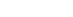then

Sum = 1 + 2 + 3 + 4 + 5 + 6 + 7 + 8 + 9 + 10 = 55

### Program Code – Sum of First N Natural Numbers

/* Program for sum of First N Natural Numbers */
#include <stdio.h>
#include <stdlib.h>
main() {
int num,i,sum;
sum = 0; /* read the number */
printf("Enter number to sum:");
scanf("%d",&num);
for(i=1;i<=num;i++)     {
sum = sum + i;
}
printf("sum of %d number is %d\n",num,sum);
getch();
return 0;
}

The most important piece of code in the above example is the for loop.

for ( i = 1; i <= num; i++)
{
sum = sum + 1;
}

The for loop starts from 1 and goes through each digit between 1 and num because variable i is incremented with each iteration ( i++).  The value of num is user input and it can be a large number.

The value of i is added to variable sum using the following statement. The value of sum also increments with each iteration of the variable i in the for loop.

sum = sum + i;

The final value of the sum is printed as output.

### Output

How many numbers to add?:10
------------------------
sum of 10 number is 55
------------------------

## C Program To Compute Nth Fibonacci Number

The program computes Nth Fibonacci number using a technique called recursion and prints the results. A recursion is the ability of a procedure or function to call itself several times.

You can write the program without recursion, but computer science students learn Fibonacci series as an introduction to recursion or recurrence relations. It is a topic of higher importance in both mathematics and computer science.

We compiled the program using Dev-C++ version 4 compiler on a Windows 7 64-bit system, but you can also compile the program using another standard C compiler such as Turbo C++ 3 with modified source code. The choice of compiler is up to the programmer.

You should learn the following C programming concepts before trying the example program.

### Problem Definition

In mathematics, the whole number means all positive numbers starting with 0. Any term in Fibonacci series takes a sum of previous two terms. The formula for the nth term of Fibonacci series is given below.

\begin{aligned}
& f_{n} = f_{n-1} + f_{n-2}
\end{aligned}

The first few terms of the series are as follows

\begin{aligned}
&0, 1, 1, 2, 3, 5, 8, 13, 21, 34, \cdots
\end{aligned}

### Program Code – Fibonacci Series

/* Program to calculate Fibonacci Series */
#include < stdio.h >
#include < stdlib.h >
main ()
{
int n;
void fib ();
printf ("Number of terms to be generated?");
scanf ("%d", & n);
printf ("%d", n);
printf ("\n\n Fibonacci sequence up to %d terms :\n\n");
fib(n);
printf("\n");
system("PAUSE");
return 0;
}

void fib(int n)
{
static long int f1 = 0, f2 = 1, sum;
if (n > 0)
{
sum = f1 + f2;
f1 = f2;
printf("%5d", sum);
f2 = sum;
fib(n - 1);
}
}

### Output

Number of terms to be generated?:8
Fibnacci sequence upto 8 terms :
-------------------------------------------------------
1     2     3     5     8     13    21     34
-------------------------------------------------------

## C Program to Swap Two Numbers Without a Third Variable

The program to swap two number uses the simple procedure with a fewer number of variables.The regular swapping procedure contains an extra variable called temp, a temporary variable to hold values in between the swap task. This program does not use any temp variable and achieve the same results.

We wrote the program with Dev-C++ version 4 compiler installed on a Windows 7 64-bit PC.  If you may try another standard C compiler, like Turbo C++ 3.0, then modify the source code according to the compiler specifications.

Learn following c programming concepts before you try this example program. If you know the concepts already, then continue reading the article.

### Problem Definition

The following code shows the common method to swap two integer numbers within a C program. As mentioned earlier, it uses a temp variable. The temp is assigned the value of variable a and a is assigned the value of variable b.

\begin{aligned}
&temp = 10\\ \\
&a = 20
\end{aligned}

In the next step, b is assigned the value of temp to complete the swap procedure.

\begin{aligned}
&b = temp;\\ \\
&b \hspace{2mm} is \hspace{2mm} 10 \hspace{2mm}now.
\end{aligned}

Code For Swap Using A Temp Variable

a = 10
b = 20
/* Store the value of variable a into temp */
temp = a;
/* Value of b is assigned to a */
a = b;
/* Value of temp is assigned to b*/
b = temp; 

### Program Code – Swap Numbers without Third Variable

/* Program to swap two numbers without a third variable */

#include <stdio.h>
#include <conio.h>
int main()
{
int a,b; int i;
printf("Enter value for A:");
scanf("%d",&a);
printf("Enter value for B:");
scanf("%d",&b);
/*Swap the Variables with out a third variable*/
a = a + b;
b = a - b;
a = a - b;
/* Print the result */
for(i=0;i<35;i++)
printf("_");printf("\n\n");
printf("A = %d B = %d\n\n",a,b);
for(i=0;i<35;i++)
printf("_");printf("\n\n");
getch();
return 0;
}

The above source code shows efficient use of arithmetic operators to swap the number and moreover, it is faster because there is no unnecessary assignments of variables.

### Output

Enter value for A:24
Enter value for B:44
----------------------------------------
A = 44 B = 24
----------------------------------------